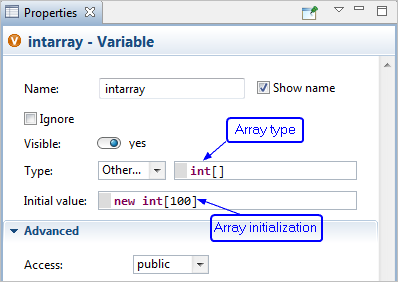AnyLogic

# Arrays

Java arrays are containers with linear storage of fixed size. To create an array you should declare a variable of array type and initialize it with a new array, like this:

``int[] intarray = new int; //array of 100 integer numbers``

Array type is composed of the element type followed by square brackets, e.g. int[], double[], String[], Agent[]. The size of the array is not a part of its type. Allocation of the actual storage space (memory) for the array elements is done by the expression new int, and this is where the size is defined. Note that unless you initialize the array with the allocated storage, it will be equal to null, and you will not be able to access its elements.

A graphical declaration of the same array of 100 integers will look like the Figure. You should use a Variable or Parameter object, choose Other for Type and enter the array type in the field on the right.

Do not be confused by the checkbox Array in the properties of Parameter: that checkbox sets the type of parameter to System Dynamics HyperArray and not to Java array.Array variable declared graphically

All elements of the array initialized that way will be set to 0 (for numeric element type), false for boolean type and null for all classes including String. Another option is to explicitly provide initial values for all array elements. The syntax is the following:

``int[] intarray = new int[] { 13, x-3, -15, 0, max{ a, 100 ) };``

The size of the array is then defined by the number of expressions in braces. To obtain the size of an existing array you should write <array name>.length, for example:

``intarray.length``

The ith element of an array (remember that array indexes are 0-based) can be accessed as:

``intarray[i - 1]``

Iteration over array elements is done by index. The following loop increments each element of the array:

``````for(int i=0; i<intarray.length; i++) {
intarray[i]++;
}``````

Java also supports a simpler form of the “for” loop for arrays. The following code calculates the sum of the array elements:

``````int sum = 0;
for(int element : intarray) {
sum+=element;
}``````

Arrays can be multidimensional. This piece of code creates a two-dimensional array of double values and initializes it in a loop:

``````double[][] doubleArray = new double;
for(int i=0; i < doublearray.length; i++) {
for(int j=0; j < doubleArray[i].length; j++ ) {
doubleArray[i][j] = i * j;
}
}``````

You can think of a multidimensional array as “array of arrays”. The initialized as new double is array of 10 arrays of 20 double values each. Notice that doubleArray.length returns 10 and doubleArray[i].length returns 20.